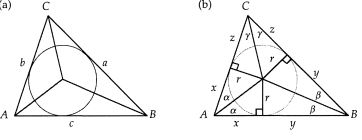D

#### Heron's formula

21 viewed last edited 3 years agoAnthony Schwartz
0
How do I prove the Heron's formula for the area of a triangle with sides a, b \text{ and } c and s= \frac{a+b+c}{2} : A = \sqrt{s (s-a)(s-b)(s-c)} ? I found the Wikipedia article (https://en.wikipedia.org/wiki/Heron%27s_formula) giving the proof, but can anybody provide a good intuitive proof?Sangeetha Pulapaka
0Let \triangle ABC be a triangle with sides a, b, c as in figure (a) and bisect each angle to locate the center of the incircle. Extending an inradius to each side partitions the triangle into six smaller right triangles with side lengths indicated as in (b). Note that the semiperimeter satisfies s = x+y+z = x+a = y+b = z+c. There are two lemmas from which Heron's formula readily follows. Lemma 1: The area k of a triangle is equal to the product of its inradius and perimeter. Lemma 2: If \alpha \beta \gamma are any positive angles such that \alpha+\beta+\gamma = \frac{\pi}{2} then tan \alpha tan \beta+ tan \beta tan \gamma + tan \gamma tan \alpha = 1. Heron's formula is The area of K of a triangle with sides of length a , b and c is given by K = \sqrt{s(s-a)(s-b)(s-c)} where S = (a+b+c)/2. For proving this we apply Lemma 2 to the angles labeled as \alpha, \beta, \gamma in figure (b) which yields 1 = tan\alpha tan\beta + tan\beta tan\gamma + tan\gamma tan\alpha = \frac{r}{x} \frac{r}{y} +\frac{r}{y} \frac{r}{z} + \frac{r}{z} \frac{r}{x} = \frac{{r}{^2}(x+y+z)}{xyz} = \frac{{r}{^2}{s}}{xyz} where the last step follows from Lemma 1. Invoking 1 yeilds {k}{^2} = sxyz = s(s-a)(s-b)(s-c) which completes the proof.